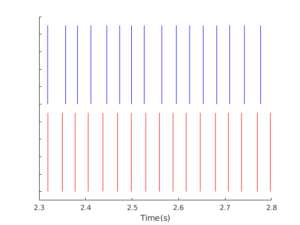# Comparing Spike Trains Using Coincidence

Git repository: https://bitbucket.org/erikjohnson24/eriksresearchcode

A problem that comes up again and again in neuroscience research is the comparison of spike-trains.

What is a spike-train? When looking at the membrane voltage along a neuron’s axon, you’ll see rapid depolarizations of the voltage termed action potentials or spikes. Neurons transmit sequences of spikes down their axons, called a spike-train. Since all the action potentials for a given neuron are (more or less) identical, such a signal can be represented as$\sum_i \delta(t-t_i)$

where$\delta(t)$ is the Dirac delta function. In such a signal, information must be encoded in the time of the spikes.

How can one best compare two spike-trains? Someday I’d like to write up a full discussion of the problem, but here I’m just going to describe one metric, spike-time coincidence, and link some code.

Imagine two spike trains, with their rasters plotted below.A raster plot of two simulated spike trains, one in blue, one in red. How similar are these two?

Are these similar? One intuitive idea is to see how often the spikes “line up”, and count the number of spikes which line up, or are coincident. This is the basic idea of the spike-time coincidence metric. Count up the number of spikes in the two spike trains which fall within a window of$\Delta$ seconds of each other.

This is then normalized so that 1 corresponds to a perfect match between the two spike trains (the exact same number of spikes with all spikes coincident) and 0 corresponds to the expected number of coincidences for a Poisson process with similar spike-rate. The coincidence can go negative (a very bad match indeed). I believe this metric was introduced by Kistler, Gerstner, and Hemmen in 1997, modified from work by Joeken and Schwegler. Since then, it has been used quite a bit, notably in the INCF Spike-Time Prediction Competition (and less notably in the work from my lab).

The spike-time coincidence is defined as$\Gamma = \frac{N_{\text{coin}}-E[N_{\text{coin}}]}{N_{\text{train}_1}+N_{\text{train}_2}} \frac{2}{1-2\nu \Delta}$

where$\Delta$ is the coincidence window,$N_{\text{coin}}$ is the number of coincidences,$E[N_{\text{coin}}]$ is the number of expected coincidences from a Poisson point process with the same rate as the second spike-train,$N_{\text{train}_1}$ is the number of spikes in the first spike-train,$N_{\text{train}_2}$ is the number of spikes in the second spike-train,  and$\nu$ is the spike rate of the second spike-train.

The key advantages of this metric are the intuition of counting which spikes “line up” and the fact that each spike time is taken into consideration. The only major assumption that has to be made is the window size$\Delta$. This metric is a great way to compare spike-trains directly to say something like “X% of spikes were predicted with a Yms accuracy using our model.” The key downside, in my opinion, is the difficulty of mathematic analysis. The coincidence is nonlinear in the spike-times and has no particularly redeeming qualities from an optimization perspective.

Code to calculate coincidence is given here:

https://bitbucket.org/erikjohnson24/eriksresearchcode/src/master/neural/coin_calc.m

There is also testing code, where you can vary the jitter to see the change in coincidence.

Someday, I will hopefully find a time to do a more detailed comparison of spike-train metrics!

Posted on